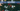# Binary Search Tree (BST) Data Structure

August 17, 2020A Binary Search tree (BST) is a data structure which has two children nodes attached to it, called left and right node. There is a special relation between the parent and left-right child.

Its a Binary Tree, but have relation maong values between parent and children as mentioned above.

Few Basics if Binary Search Tree:

• Parent node value is greater than its left child
• Right child has the greater value than parent
• So, left node is the one having least value
• Parent node can have minimum zero children or 2 children maximum.

## Some Considerations

This data structure can be used at any place where you want to represent upto 2 children. This specialized version of Binary Tree is very helpful when you want to search nodes among the complete tree.

You can decide from the root node itself, whether your value to be search lies either on left side or right side.

## Basic Data Structure

Lets look at the basic data structure to denote a Binary Tree.

``````public class Node {
public int data;
public Node left;
public Node right;

public Node(int data) {
this.data = data;
this.left = null;
this.right = null;
}
}``````

Above code is in Java. A tree node has three things:

1. The data (It can be any data type, I have taken integer for simplicity)
2. A pointer/reference to left child
3. A pointer/reference to right child

Note the data type is same for left and right node.

Lets take a look at a representation of a Binary Tree:

``````        50
/    \
20      90
/    \      \
10     30      100``````

## Create Sample Binary Search Tree with code

Lets see a small code on how we can create above tree with the `class Node` data structure.

``````public Node buildSampleTree() {
Node root = new Node(50);
root.left = new Node(20);
root.right = new Node(90);

root.left.left = new Node(10);
root.left.right = new Node(30);

root.right.right = new Node(100);

return root;
}``````